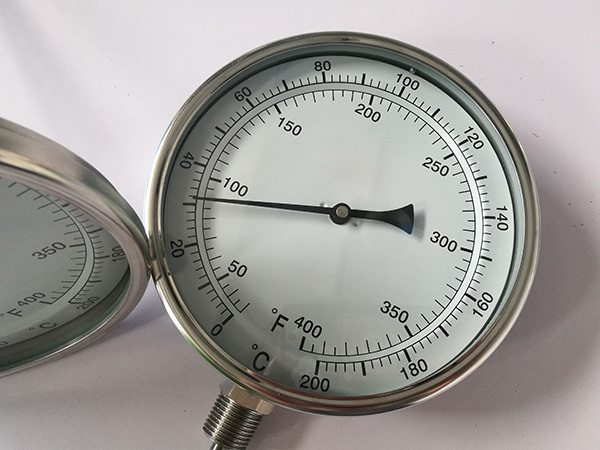How to Calculate Precision？

# How to Calculate Precision？

Summary

In a laboratory setting, accuracy is an indication of how close your measurements are to the actual, true measure of something, whereas precision is an indication of how close your measurements are to one another.

The words"precision" and "accuracy" are thrown around a lot, often without specific regard to their actual meaning. When you are throwing darts at a bullseye, you are aiming for the center. Whether or not you can hit the bullseye indicates how accurate you are; the closeness of the darts to one another indicates how precise you are. In a laboratory setting, accuracy is an indication of how close your measurements are to the actual, true measure of something, whereas precision is an indication of how close your measurements are to one another.Findthe Average

The average, or mean, of a set of values is the typical value in that dataset,calculated by adding all of the measured values and then dividing by the number of values you measured. For example, to measure the height of a friend, use a tape measure to measure her height five times and record these values. Add all of the height measurements together and divide by five -- the number of measurements you took. This will give you your mean height measurement.

Find the Variance

The variance of a dataset tells you how far your measurements are from the mean value of the dataset, often referred to as the variation around the mean. To calculate how much each of your values deviates from the mean value, take the five height measurements that you recorded and subtract the mean value of the dataset from each one. To calculate the variance within your dataset, square each of the five deviations and add them all together. Divide that number by four -- the number of height values you measured minus one, to obtain the variance.

Find the Standard Deviation

In order to find out how much each of your measured height values vary from one another, in other words how spread out your datapoints are, you will calculate standard deviation. Standard deviation is a deceptively simple calculation: it is just the square root of the variance! The standard deviation is calculated using all five of your measurements and represents the precision of a single typical measurement.

Find the Precision

The standard error of the mean is the measure of how close your data points are to the true mean, and represents your precision. In order to calculate the standard error of the mean, you take the standard deviation from the set offive measurements of your friend's height and divide it by the square root of the number of samples -- which in this case is five.